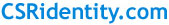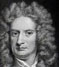CSRidentitySir Isaac Newton PRS (25 December 1642 – 20 March 1726) was an English physicist and mathematician (described in his own day as a "natural philosopher") who is widely recognised as one of the most influential scientists of all time and a key figure in the scientific revolution. Newton's Principia formulated the laws of motion and universal gravitation, which dominated scientists' view of the physical universe for the next three centuries. By deriving Kepler's laws of planetary motion from his mathematical description of gravity, and then using the same principles to account for the trajectories of comets, the tides, the precession of the equinoxes, and other phenomena, Newton removed the last doubts about the validity of the heliocentric model of the Solar System. This work also demonstrated that the motion of objects on Earth and of celestial bodies could be described by the same principles. Newton built the first practical reflecting telescope and developed a theory of colour based on the observation that a prism decomposes white light into the many colours of the visible spectrum. He formulated an empirical law of cooling, studied the speed of sound, and introduced the notion of a Newtonian fluid. In addition to his work on calculus, as a mathematician Newton contributed to the study of power series, generalised the binomial theorem to non-integer exponents, developed a method for approximating the roots of a function, and classified most of the cubic plane curves.